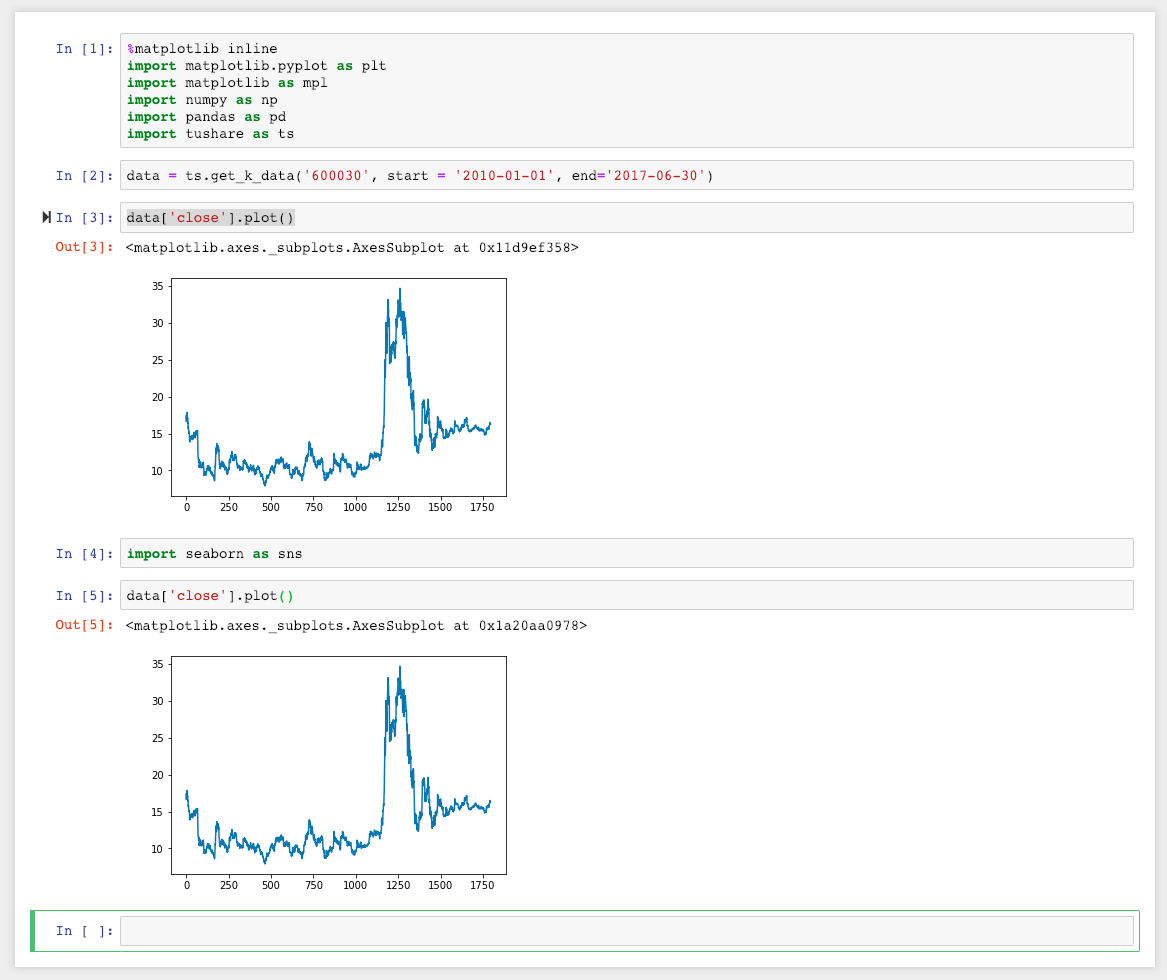Jupyter notebook中import seaborn无效果

``````%matplotlib inline
import matplotlib.pyplot as plt
import matplotlib as mpl
import numpy as np
import pandas as pd
import tushare as ts
``````
``````data = ts.get_k_data('600030', start = '2010-01-01', end='2017-06-30')
``````
``````data['close'].plot()
``````
``````import seaborn as sns
``````
``````data['close'].plot()
``````2个回答

import seaborn as sns
sns.set()
data['close'].plot()jupyter notebook 目录.

jupyter 能在浏览器打开，看得见目录，但是就没法写代码，也查看不了，新建一个PYTHON3文件时或者打开都显示500 error。 我是新建一个再加载了tensorflow和JUPYTER，在那里打开的，但是在base（root）里面就没有问题

jupyter 使用 %matplotlib notebook 绘出交互式图形后，为什么图形的下一行，按Enter键不能进入代码格式

jupyter notebook 使用 %matplotlib notebook 绘出交互式图形后，为什么图形的下一行，按Enter键不能进入代码格式，输入代码 各位大佬，可有解决办法 ``` import pandas as pd import seaborn as sns %matplotlib notebook sns.set() tips = sns.load_dataset('tips') sns.distplot(tips['total_bill'], bins=16, color='purple') ``` ![图片说明](https://img-ask.csdn.net/upload/201906/19/1560945613_791251.png)

Seaborn的使用问题（倒数第二行代码报错）

import numpy as np import pandas as pd import seaborn as sns import matplotlib.pyplot as plt import statsmodels.api as sm from statsmodels.formula.api import ols,glm #将数据集读入到pandas数据框中 wine = pd.read_csv('winequality-both.csv',sep=',',header=0) wine.columns = wine.columns.str.replace(' ','_') print(wine.head()) #显示所有变量的描述性统计量 print(wine.describe()) #找出唯一值 print(sorted(wine.quality.unique())) #计算值的概率 print(wine.quality.value_counts()) #按照葡萄酒类型显示质量的描述性统计量 print(wine.groupby('type')[['quality']].describe().unstack('type')) #按照葡萄酒的类型显示质量的特定分位数值 print(wine.groupby('type')[['quality']].quantile([0.25,0.75]).unstack('type')) #按照葡萄酒类型查看质量分布 red_wine = wine.loc[wine['type']=='red','quality'] white_wine = wine.loc[wine['type']=='white','quality'] sns.set_style("dark") print(sns.distplot(red_wine,norm_hist=True,kde=False,color="red",label="Red Wine")) print(sns.distplot(white_wine,norm_hist=True,kde=False,color="white",label="White Wine")) sns.axlabel("Quality Score","Density") plt.title("Distribution of Quality by Wine Type") plt.legend() plt.show() #检验红葡萄酒和白葡萄酒的平均质量是否有所不同 print(wine.groupby(['type'])[['quality']].agg(['std']) tstat, pvalue, df = sm.stats.ttest_ind(red_wine,white_wine) print('tstat:%.3f pvalue:%.4f' % (tstat,pvalue)) ![图片说明](https://img-ask.csdn.net/upload/201811/10/1541840583_803202.png)

pip安装成功 ``` C:\Users\Administrator>pip install seaborn-0.7.0-py2.py3-none-any.whl Processing c:\users\administrator\seaborn-0.7.0-py2.py3-none-any.whl Installing collected packages: seaborn Successfully installed seaborn-0.7.0 ``` 导入包时，报错 ``` C:\Users\Administrator>python Python 2.7.11 (v2.7.11:6d1b6a68f775, Dec 5 2015, 20:32:19) [MSC v.1500 32 bit ( Intel)] on win32 Type "help", "copyright", "credits" or "license" for more information. >>> import seaborn Traceback (most recent call last): File "<stdin>", line 1, in <module> File "D:\Python27\lib\site-packages\seaborn\__init__.py", line 1, in <module> from .rcmod import * File "D:\Python27\lib\site-packages\seaborn\rcmod.py", line 8, in <module> from . import palettes File "D:\Python27\lib\site-packages\seaborn\palettes.py", line 12, in <module> from .utils import desaturate, set_hls_values, get_color_cycle File "D:\Python27\lib\site-packages\seaborn\utils.py", line 8, in <module> from scipy import stats File "D:\Python27\lib\site-packages\scipy\stats\__init__.py", line 338, in <mo dule> from .stats import * File "D:\Python27\lib\site-packages\scipy\stats\stats.py", line 180, in <modul e> import scipy.special as special File "D:\Python27\lib\site-packages\scipy\special\__init__.py", line 627, in < module> from ._ufuncs import * ImportError: DLL load failed: 找不到指定的模块。 >>> ``` python版本2.7 我的系统是win7系统 64位 ![图片说明](https://img-ask.csdn.net/upload/201604/15/1460714570_426118.png)

import matplotlib.pyplot as plt报错

conda list里面已经安装了seaborn，但是pycharm里面无法使用，求助怎么解决

seaborn画图为什么回归线不拟合散点分布？

Python seaborn factorplot 图表意义

Python seaborn factorplot 图表有何含义？如何计算？ 如plot 如何计算得出？plot 上的线如何计算，有何含义？

python 做热图的时候，总是遇到Error

python用plotly散点图分区显示不同颜色问题

<div class="post-text" itemprop="text"> <p>I have a python program that starts with: </p> <pre><code>from optparse import OptionParser import math #import wx import os import numpy as np import matplotlib.pyplot as plt from pylab import * from numpy import * import scipy as scipy from scipy import * from scipy import constants import scipy.signal as signal import matplotlib.pyplot as plt </code></pre> <p>It gives me error when I try to open it with php. I have googled and apparently if I do this before importing pylab or pyplot:</p> <pre><code>import matplotlib matplotlib.use('Agg') import matplotlib.pyplot as plt </code></pre> <p>Problem should be solved. But the error I get is:</p> <pre><code> /usr/lib/pymodules/python2.7/matplotlib/__init__.py:923: UserWarning: This call to matplotlib.use() has no effect because the the backend has already been chosen; matplotlib.use() must be called *before* pylab, matplotlib.pyplot, or matplotlib.backends is imported for the first time </code></pre> <p>Any idea what's going on??</p> </div>

``` 就是我行对train文件训练后，直接训练test.xls。请问这个应该怎么办呢，越简单越好。 import numpy as np import pandas as pd import matplotlib.pyplot as plt import seaborn as sns #from competition.ml import score %matplotlib inline %config InlineBackend.figure_format = 'retina' %config IPCompleter.greedy = True %config IPCompleter.use_jedi = True pd.options.display.max_colwidth = 100 plt.rcParams['figure.figsize'] = (12, 8) train_path = r'C:\Users\MyPC\Desktop\train.xls' test_path = r'C:\Users\MyPC\Desktop\test.xls' train = pd.read_excel(train_path) test = pd.read_excel(test_path) train.shape, test.shape train.info() test.info() train.head() test.head() train.describe() test.describe() from sklearn.ensemble import ExtraTreesClassifier model=ExtraTreesClassifier() trainX=train[['X1','X2','X3','X4','X5','X6','X7','X8','X9','X10','X11','X12','X13','X14','X15','X16','X17','X18','X19','X20','X21','X22','X23']] trainY=train['Y'] testX=train[['X1','X2','X3','X4','X5','X6','X7','X8','X9','X10','X11','X12','X13','X14','X15','X16','X17','X18','X19','X20','X21','X22','X23']] testY=train['Y'] model.fit(trainX,trainY) y_pred =model.predict(testX)#######预测 np.savetxt(r'C:\Users\MyPC\Desktop\predict.csv', y_pred) #score(y_pred) 就是我行对train文件训练后，直接训练test.xls。请问这个应该怎么办呢，越简单越好。 ```

URLError: <urlopen error [WinError 10054] 远程主机强迫关闭了一个现有的连接？

“亚马逊丛林里的蝴蝶扇动几下翅膀就可能引起两周后美国德州的一次飓风……” 这句人人皆知的话最初用来描述非线性系统中微小参数的变化所引起的系统极大变化。 而在更长的时间尺度内，我们所生活的这个世界就是这样一个异常复杂的非线性系统…… 水泥、穹顶、透视——关于时间与技艺的蝴蝶效应 公元前3000年，古埃及人将尼罗河中挖出的泥浆与纳特龙盐湖中的矿物盐混合，再掺入煅烧石灰石制成的石灰，由此得来了人...

C++11：一些微小的变化（新的数据类型、template表达式内的空格、nullptr、std::nullptr_t）

HashMap底层实现原理，红黑树，B+树，B树的结构原理 Spring的AOP和IOC是什么？它们常见的使用场景有哪些？Spring事务，事务的属性，传播行为，数据库隔离级别 Spring和SpringMVC，MyBatis以及SpringBoot的注解分别有哪些？SpringMVC的工作原理，SpringBoot框架的优点，MyBatis框架的优点 SpringCould组件有哪些，他们...

【阿里P6面经】二本，curd两年，疯狂复习，拿下阿里offer

《经典算法案例》01-08：如何使用质数设计扫雷（Minesweeper）游戏

《Oracle Java SE编程自学与面试指南》最佳学习路线图（2020最新版）